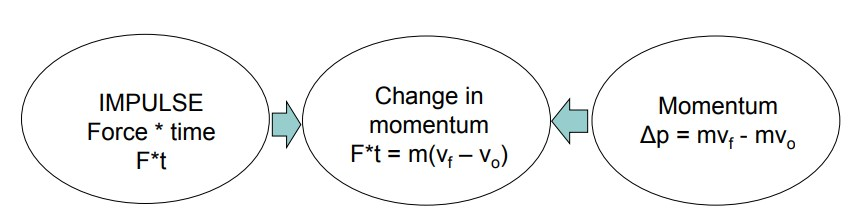# Impulse Calculator

Use our Impulse Calculator a free online tool to calculate the the change in momentum of an object. Enter the values of mass and velocity change to calculate impulse force.

## Impulse Formula

The impulse is defined as the change in the momentum of an object. So, if you know the mass and change in velocity or mass of an object and its initial and final velocity; then you can calculate impulse of an object by the formula:

Impulse (I) = m * Δ v

The units of Impulse is kg.m/s

If you know the force (F) acting on an object and the duration of the force (t); then use the following formula to calculate impulse force:

Impulse (J) = Applied Force (F) x Elapsed Time (t) = F * t

Here, Unit of Impulsive Force is Newtons.seconds (N.s)### What is momentum?

An object’s momentum is the product of its mass and velocity. An object can have a large momentum by having a large mass or a large velocity. Momentum is a vector quantity.

#### Impulse and Change in Momentum of an Object

According to Newton’s 2nd Law: The application of a net force on an object will result in the object accelerating (aka changing velocity)

F = ma = m(Δv/t) = m(vf – vo)/t, but
Ft = mvf – mvo = pf – po thus
Ft (or impulse) = change in momentum or Ft = mΔv
F = mΔv/t = m(vf – v0)/t (Newton’s 2nd law in momentum terms; vf is final velocity, v0 is initial velocity)

#### How Impulse, Momentum and Change in Momentum are Interrelated### Spreading Knowledge Across the World

USA - United States of America  Canada  United Kingdom  Australia  New Zealand  South America  Brazil  Portugal  Netherland  South Africa  Ethiopia  Zambia  Singapore  Malaysia  India  China  UAE - Saudi Arabia  Qatar  Oman  Kuwait  Bahrain  Dubai  Israil  England  Scotland  Norway  Ireland  Denmark  France  Spain  Poland  and  many more....